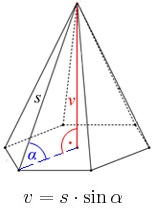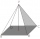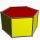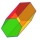# Hexagonal pyramid

Regular hexagonal pyramid has dimensions: length edge of the base a = 1.8 dm and the height of the pyramid = 2.4 dm. Calculate the surface area and volume of a pyramid.

S =  23.8716 dm2
V =  6.7342 dm3

### Step-by-step explanation:Did you find an error or inaccuracy? Feel free to write us. Thank you!Tips to related online calculators
Pythagorean theorem is the base for the right triangle calculator.
Do you want to convert length units?
Tip: Our volume units converter will help you with the conversion of volume units.

#### You need to know the following knowledge to solve this word math problem:

We encourage you to watch this tutorial video on this math problem:

## Related math problems and questions:

• Hexagonal pyramidCalculate the volume and the surface of a regular hexagonal pyramid with a base edge length of 3 cm and a height of 5 cm.
• Hexagonal pyramidCalculate the volume and surface area of a regular hexagonal pyramid with a base edge a = 30 m and a side edge b = 50 m.
• Hexagonal pyramidPlease calculate the height of a regular hexagonal pyramid with a base edge of 5cm and a wall height of w = 20cm. Please sketch a picture.
• Regular hexagonal pyramidCalculate the height of a regular hexagonal pyramid with a base edge of 5 cm and a wall height of w = 20cm. Sketch a picture.
• Hexaprism containerCalculate the volume and surface in the shape of a regular hexagonal prism with a height of 1.4 m with a base edge of 3dm and a corresponding height of 2.6 dm.
• Hexagonal pyramidFind the area of a shell of the regular hexagonal pyramid, if you know that its base edge is 5 cm long and the height of this pyramid is 10 cm.
• HexagonCalculate the regular hexagonal prism's surface whose base edge a = 12cm and side edge b = 3 dm.
• Hexagonal pyramidCalculate the surface area of a regular hexagonal pyramid with a base inscribed in a circle with a radius of 8 cm and a height of 20 cm.
• Tetrahedral pyramidWhat is the surface of a regular tetrahedral (four-sided) pyramid if the base edge a=16 and height v=16?
• Hexagonal pyramidThe base of the pyramid is a regular hexagon, which can be circumscribed in a circle with a radius of 1 meter. Calculate the volume of a pyramid 2.5 meters high.
• Hexagonal pyramidFind the volume of a regular hexagonal pyramid, the base edge of which is 12 cm long and the side edge 20 cm.
• Pentagonal pyramidThe height of a regular pentagonal pyramid is as long as the edge of the base, 20 cm. Calculate the volume and surface area of the pyramid.A regular quadrilateral pyramid has a volume of 24 dm3 and a base edge a = 4 dm. Calculate: a/height of the pyramid b/sidewall height c/surface of the pyramidThe pyramid with a rectangular base measuring 6 dm and 8 dm has a side edge of length 13 dm. Calculate the surface area and volume of this pyramid.Calculate the volume and surface area of a regular triangular pyramid with height equal to the base edge 10 cm length.Calculate the volume and surface of 4 sides regular pyramid whose base edge is 4 cm long. The angle from the plane of the sidewall and base plane is 60 degrees.In a regular 4-sided pyramid, the side edge forms an angle of 55° with the base's diagonal. The length of the side edge is eight meters. Calculate the surface area and volume of the pyramid.